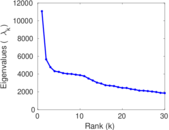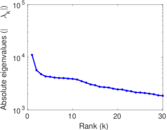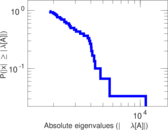# Discogs

Discogs (short for "discographies") is a large online music database that provides information about audio records including information about artists, labels and release details. This bipartite network represents the affiliations between artists and labels. The left nodes represent artists and the right nodes represent labels. Each edge represents one release made by an artist with a label.

 Code `Dl` Internal name `discogs_affiliation` Name Discogs Data source http://www.discogs.com/ AvailabilityDataset is available for download Consistency checkDataset passed all tests Category Affiliation network Node meaning Artist, label Edge meaning Affiliation Network formatBipartite, undirected Edge typeUnweighted, multiple edges

## Statistics

 Size n = 2,025,594 Left size n1 = 1,754,823 Right size n2 = 270,771 Volume m = 14,414,659 Unique edge count m̿ = 5,302,276 Wedge count s = 13,051,812,272 Claw count z = 208,086,141,206,057 Cross count x = 3,769,613,215,333,388,288 Square count q = 3,261,758,502 4-Tour count T4 = 78,312,501,992 Maximum degree dmax = 257,360 Maximum left degree d1max = 257,360 Maximum right degree d2max = 199,896 Average degree d = 14.232 5 Average left degree d1 = 8.214 31 Average right degree d2 = 53.235 6 Fill p = 1.115 90 × 10−5 Average edge multiplicity m̃ = 2.718 58 Size of LCC N = 1,924,972 Diameter δ = 22 50-Percentile effective diameter δ0.5 = 3.806 12 90-Percentile effective diameter δ0.9 = 5.753 49 Median distance δM = 4 Mean distance δm = 4.678 53 Gini coefficient G = 0.861 672 Balanced inequality ratio P = 0.138 158 Left balanced inequality ratio P1 = 0.186 605 Right balanced inequality ratio P2 = 0.121 320 Relative edge distribution entropy Her = 0.857 945 Power law exponent γ = 2.539 89 Tail power law exponent γt = 2.321 00 Tail power law exponent with p γ3 = 2.321 00 p-value p = 0.049 000 0 Left tail power law exponent with p γ3,1 = 2.211 00 Left p-value p1 = 0.000 00 Right tail power law exponent with p γ3,2 = 2.111 00 Right p-value p2 = 0.000 00 Degree assortativity ρ = −0.039 421 6 Degree assortativity p-value pρ = 0.000 00 Spectral norm α = 11,073.1 Spectral separation |λ1[A] / λ2[A]| = 1.955 97 Controllability C = 1,528,426 Relative controllability Cr = 0.754 557

## Plots

### Degree distribution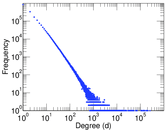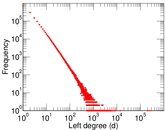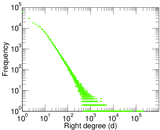### Cumulative degree distribution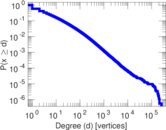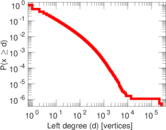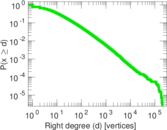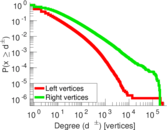### Lorenz curve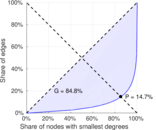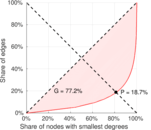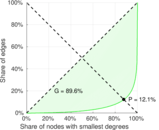### Spectral distribution of the adjacency matrix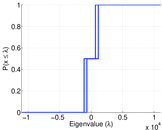### Spectral distribution of the normalized adjacency matrix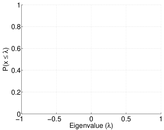### Spectral distribution of the Laplacian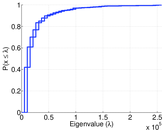### Spectral graph drawing based on the adjacency matrix### Spectral graph drawing based on the Laplacian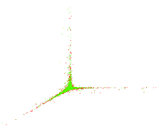### Spectral graph drawing based on the normalized adjacency matrix### Zipf plot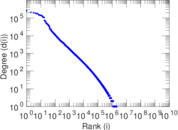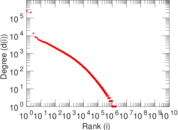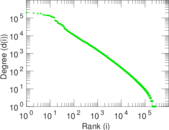### Hop distribution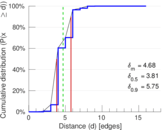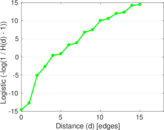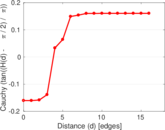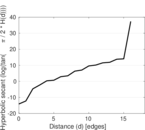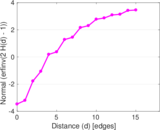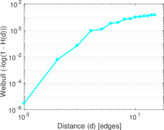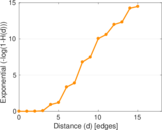### Edge weight/multiplicity distribution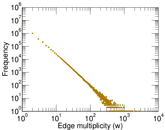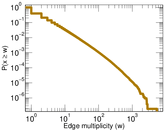### Matrix decompositions plots